﻿ 岩石渗透率各向异性评价新方法

# 岩石渗透率各向异性评价新方法A New Method to Evaluate the Anisotropy of Rock Permeability

Abstract: The permeability of rock is a typical vector, and more and more attention is paid to the testing and evaluation methods of the anisotropy of permeability. Based on traditional permeability anisotropy, evaluation methods cannot fully reflect extreme value point effect, physical meaning is not clear, is not convenient to contrast between the core and classify faults. In order to express the characteristics of anisotropy of permeability of reservoir rock more clearly, a new method of permeability evaluation is put forward based on the study of previous permeability evaluation methods of reservoir rock and the permeability test of reservoir rock of different lithology. The geometric average of the three-dimensional permeability is used to represent the apparent permeability of the core, the relative relationship between the anisotropic permeability and the ratio of the geometric average to the arithmetic average of the three-dimensional permeability is used to quantitatively describe the degree of anisotropy. The results show that the new method can scientifically and comprehensively evaluate the anisotropy of rock permeability.

1. 引言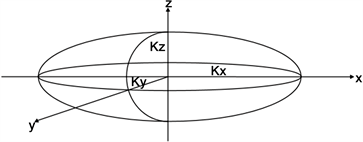Figure 1. Ellipsoid diagram of vector permeability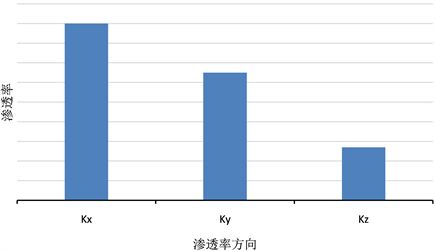Figure 2. Schematic diagram of permeability anisotropy

$\stackrel{¯}{K}=\frac{{K}_{x}+{K}_{y}+{K}_{z}}{3}$ (1)

${\delta }_{1}={K}_{i}/{K}_{j}$ (2)

${\delta }_{2}=\sqrt{\left({\left(\frac{{K}_{x}-\stackrel{¯}{K}}{\stackrel{¯}{K}}\right)}^{2}+{\left(\frac{{K}_{y}-\stackrel{¯}{K}}{\stackrel{¯}{K}}\right)}^{2}+{\left(\frac{{K}_{z}-\stackrel{¯}{K}}{\stackrel{¯}{K}}\right)}^{2}\right)/3}$ (3)

2. 描述岩石渗透率方向性大小的特征值

${K}_{a}=\sqrt{{K}_{x}{K}_{y}{K}_{z}}$ (4)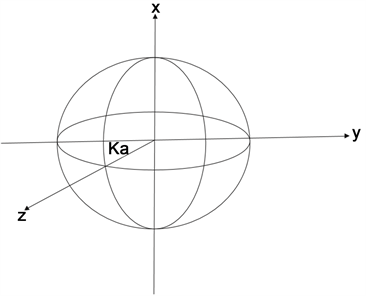Figure 3. Sphere of radius of characteristic permeability Ka

3. 三角网状图法描述储层渗透率方向性

${P}_{x}=\frac{{K}_{x}}{{K}_{x}+{K}_{y}+{K}_{z}}×100%$

${P}_{y}=\frac{{K}_{y}}{{K}_{x}+{K}_{y}+{K}_{z}}×100%$ (5)

${P}_{z}=\frac{{K}_{z}}{{K}_{x}+{K}_{y}+{K}_{z}}×100%$Table 1. Permeability anisotropy triangulation and its characteristics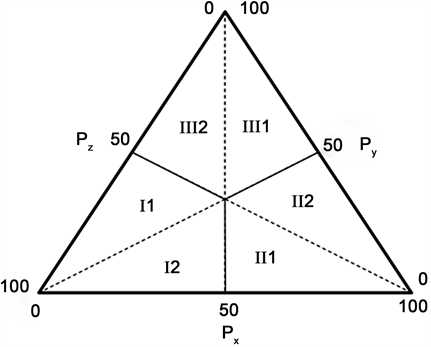Figure 4. Depicts the triangular mesh of permeability directivity of the reservoir

4. 渗透率各向异性程度定量表征

$d=\frac{\sqrt{{K}_{x}{K}_{y}{K}_{z}}}{\frac{1}{3}\left({K}_{x}+{K}_{y}+{K}_{z}\right)}$ (5)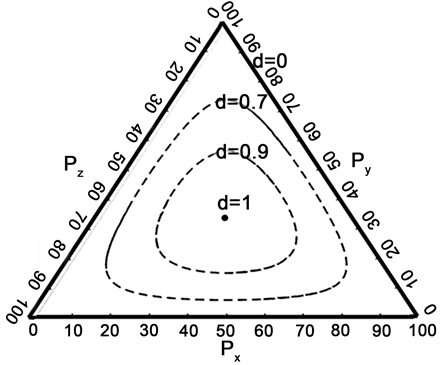Figure 5. Permeability trigonometric isogramsTable 2. Triangulation and characteristic of permeability anisotropy

5. 渗透率方向性测试分析及应用

5.1. 方向渗透率仪测量各向异性实验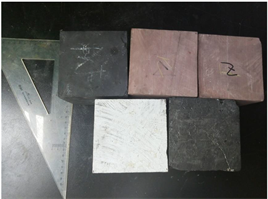Figure 6. Each rock cube sample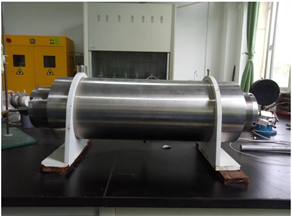Figure 7. Directional permeability core holder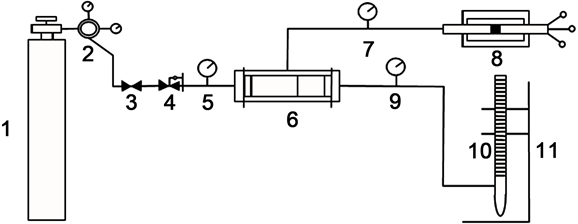1-气瓶；2-减压阀；减压阀；3-进气阀；进气阀；4-压力调节器；压力调节器；压力调节器；5，7，9-压力表；压力表；6-立方体岩心夹持器；立方体岩心夹持器；立方体岩心夹持器；立方体岩心夹持器；8-恒压泵；10-皂膜流量计； 皂膜流量计；皂膜流量计；11 -铁架台

Figure 8. Flow chart of permeability anisotropy measurement of cubic rock sampleTable 3. Data of sandstone, carbonate, shale and coal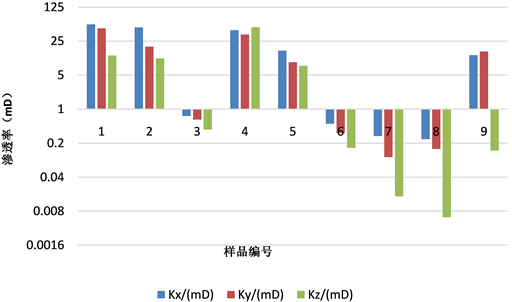Figure 9. Permeability histogram of sandstone, carbonate and shale samples

5.2. 渗透率方向性大小和非均质程度评价Table 4. Calculation table of permeability directivity and heterogeneity indexes of different samples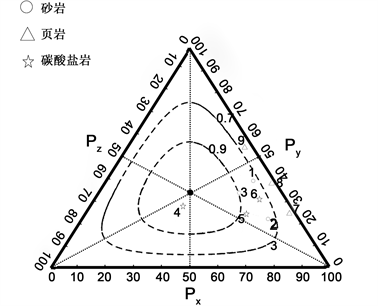Figure 10. Different sample permeability trigonometric isograms

6. 结论

1) 综合岩石的三向渗透率大小提取出描述渗透率大小的特征值，可对不同地层条件的储层或相同地层不同层位的储层渗透率大小进行比较判断；

2) 用三角图对岩石渗透率各向异性进行表征，通过对标点位置可以分析出样品渗透性特征；

3) 利用各向异性程度d和渗透率各项异性三角等值线图可以判断各样品的各向异性程度。

 Muskat, M. (1982) The Flow of Homogeneous Fluids through Porous Media. International Human Resources Development Corpration, Boston, 169-175.

 Green korn, R.A. and Johnson, C.R. (1964) Directional Permeability of Heterogeneous Anisotropic Porous Media. Society of Petroleum Engineers, 4, 115-123.

 Mast, R.F. (1963) Sedimentary Structures, Sand Shape Fabrics, and Permeability II. Journal of Geology, 71, 548-565.
https://doi.org/10.1086/626932

 Gray, D.H. and Fatt, I. (1963) The Effect Stress on Permeability of Sandstone Cores. Society of Petroleum Engineers, 3, 95-100.
https://doi.org/10.2118/531-PA

 Greenkorn, R.A., Johnson, C.R. and Shallenberger, L.K. (1964) Directional Permeability of Heterogeneous Anisotropic Porous Media. Society of Petroleum Engineers, 4, 124-132.
https://doi.org/10.2118/788-PA

 高稚文, 马志元. 柱状岩心水平渗透率张量的测量解释方法[J]. 石油学报, 1991, 12(4): 75-79.

 郭大立, 曾晓慧, 江茂泽. 计算各向异性岩心渗透率的方法研究[J]. 水动力学研究与进展, 2004, 19(1): 61-64.

 刘月田, 郭分乔, 涂彬, 等. 全岩心非均匀径向渗流各向异性渗透率测定方法[J]. 石油学报, 2005, 26(6): 66-68.

 孙东升, 李阿伟, 王红才, 等. 低渗砂岩储层渗透率各向异性规律的实验研究[J]. 地球物理学进展, 2012, 27(3): 1101-1106.

 郑新卫, 刘新华, 马天游, 等. 全直径岩心孔深分析技术[J]. 录井工程, 2013, 24(2): 27-30.

 鲍云杰, 曹婷婷, 翟怡. 岩样三向渗透率测定方法实验研究[J]. 分析仪器, 2015(1): 101-104.

 王端平, 周涌沂, 马泮光, 等. 方向性岩石渗透率的矢量特性与计算模型[J]. 岩土力学, 2005, 26(8): 1294-1297.

 王相. 各向异性岩石方向渗透率计算模型[J]. 科学技术与工程, 2013, 13(17): 5037-5039.

 王建民. 顺宁油田长21低渗砂岩储集层非均质性特征及其开发意义[J]. 石油勘探与开发, 2007, 34(2): 170-174.

 田景春, 刘伟伟, 王峰, 等. 鄂尔多斯盆地高桥地区上古生界致密砂岩储层非均质性特征[J]. 石油与天然气地质, 2014, 35(2): 183-189.

 王宇竹, 潘保芝. 渗透率各向异性测量和评价方法综述[J]. 地球物理学进展, 2017, 32(6): 2552-2559.

 陈青, 闫长辉, 蒋晓红. 塔中4号油藏渗透率分布的非均质性及渗流方向研究[J]. 长安大学学报, 2003, 25(3): 29-32.

 Ma, Y., Pan, Z., Zhong, N., et al. (2016) Experimental Study of Anisotropic Gas Permeability and Its Relationship with Fracture Structure of Longmaxi Shales, Sichuan Basin, China. Fuel, 180, 106-115.

 李传亮, 孔祥言. 渗透率参数的各向异性和不对称性的定量描述[J]. 新疆石油地质, 2000, 21(2): 128-129.

 高超, 谢凌志, 熊伦, 等. 基于特低渗砂岩覆压试验渗透率方向性特征分析[J]. 岩土力学, 2016, 37(4): 948-955.

Top